# The missing manual to SwiftUI animations

Time to get those animations working.Ever since SwiftUI came out I’ve been a big fan. Finally no more storyboards, no more duplicate nonsense of half building in code and half in a GUI.

But animations lately reminded me that there’s still a lot of less than obvious tricks you have to learn in SwiftUI. Here’s three takeaways to get you started building powerful animations in SwiftUI.

1. # The basics: there are two mechanisms for SwiftUI animations

Let’s start with a simple problem: on an 800x800 canvas, you want to animate a circle moving from one point to another. I’ll start by defining two states for the position of the circle: `xpos` and `ypos`. Then I’ll set the position of the circle with the `position`modifier.

Simple so far — here’s our preview: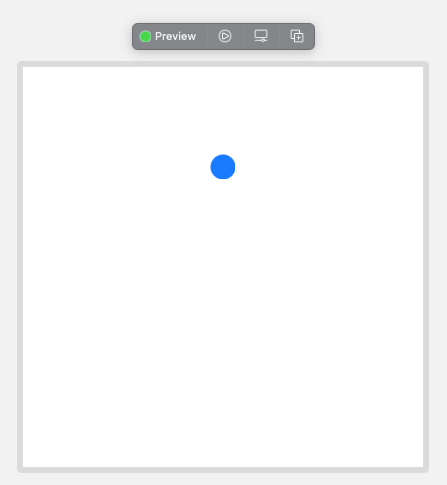Next I’ll show you both ways to animate it’s motion. The first is to use the `animation`modifier. This gets attached after the modifier that you want to animate — in this case, after the position modifier.

As part of the animation modifier, you must specify the value to monitor for changes:

``````.position(x: xpos, y: ypos)
.animation(.easeInOut(duration: 2.0), value: ypos) // Animation follows the position modifier
``````

Here we are monitoring changes to the `ypos` — this means that whenever you change `ypos` , the circle will move to the new position animated.

To trigger the change in the `ypos` , you can use for example the `onAppear` method:

``````.onAppear {
ypos = 600 // Trigger the animation to start
}
``````

Here is the complete code and the result:One problem with this approach is the `value: ypos` argument — what if you want to animate both changes in `xpos` and `ypos` ? On top of that, it’s a bit confusing to follow — we have to keep in mind that the animation modifier modifies the previous modifier (the position modifier)… ??? This can easily lead to mistakes — if you put the animation modifier directly after the Circle(), you will make it appear/disappear!

The second way to do animations is more intuitive to me — using `withAnimation` . Instead of the `.animation` modifier, we use:

``````withAnimation(.easeInOut(duration: 2.0)) {
// withAnimation tells that states modified in closure are animated
ypos = 600
}
``````

The `withAnimation` block monitors any `State` that are changed in the closure and animates them. This is the much cleaner solution to me. Now we can also freely change the `xpos` in the same block, which will also animate the position in the horizontal direction.

1. # Use animatableData to perform smarter animations along custom paths

Let’s make it more complicated — let’s animate the motion of the small blue circle around a bigger black circle: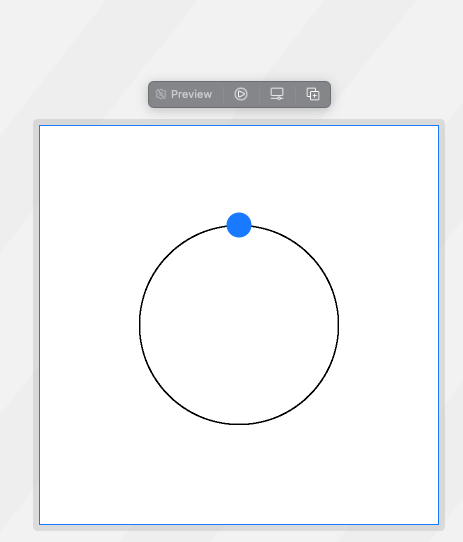To draw this I added the following to the `ZStack`:

``````Circle()
.strokeBorder(.black, lineWidth: 2)
.foregroundColor(.clear)
.position(x: 400, y: 400)
``````

Now instead of an `xpos` and `ypos` state variable, we have an `angle` around the circle:

``````@State var angle: Float = Float.pi / 2.0
``````

and the position of the smaller blue circle is given by:

``````.position(x: CGFloat(400 + radius * cos(angle)), y: CGFloat(400 - radius * sin(angle)))
``````

In the animation, we try to change the angle from the initial `pi/2` to `-pi/2` :

``````.onAppear {
withAnimation(.easeInOut(duration: 2.0)) {
// withAnimation tells that states modified in closure are animated
angle = -Float.pi / 2.0
}
}
``````

Let’s see what happens: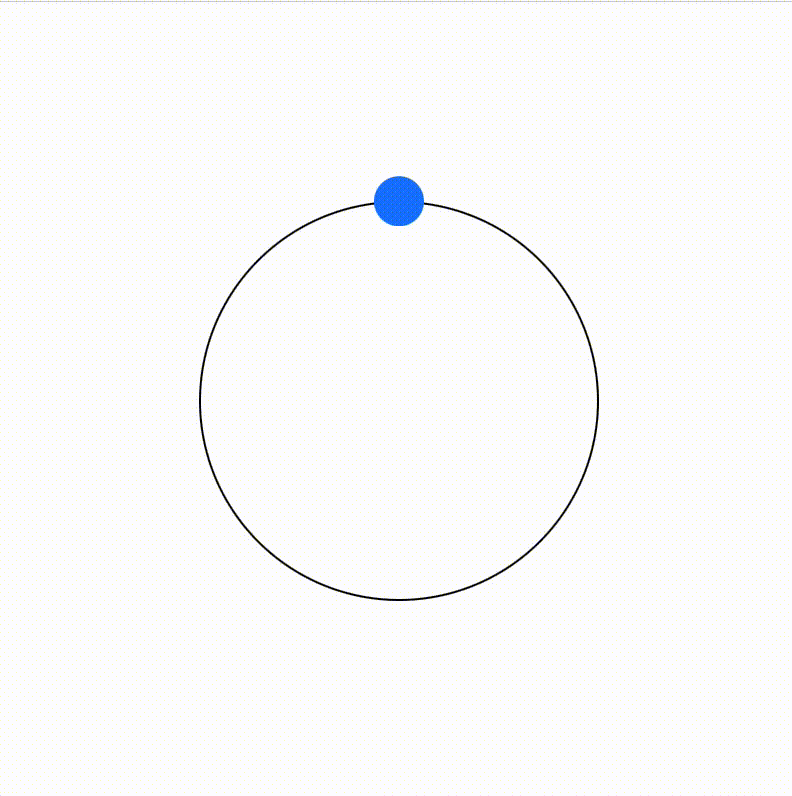… well … the theory was good, anyways.

Go around the circle!

How do we get it to go around? With the `animatableData` property.

First, we define a custom `Shape` — an animatable circle shape called `CircleWithAnimatableAngle`:

``````struct CircleWithAnimatableAngle: Shape {
var angle: Float
}
``````

The `path` function draws the circle as follows:

``````func path(in rect: CGRect) -> Path {
return Path { path in
let x = CGFloat(400 + radius * cos(angle))
let y = CGFloat(400 - radius * sin(angle))
``````
``````      path.move(to: CGPoint(x: x, y: y))
path.addEllipse(in: CGRect(x: x - 25.0, y: y - 25.0, width: 50.0, height: 50.0))
}
}
``````

Additionally, we add the `animatableData` property, which just modifies the angle:

``````var animatableData: Float {
get { angle }
set { angle = newValue }
}
``````

Finally then we can replace the `Circle` in the body view with the `CircleWithAnimatableAngle` :

``````CircleWithAnimatableAngle(angle: angle, radius: radius)
.foregroundColor(.blue)
``````

Here is the complete code and result: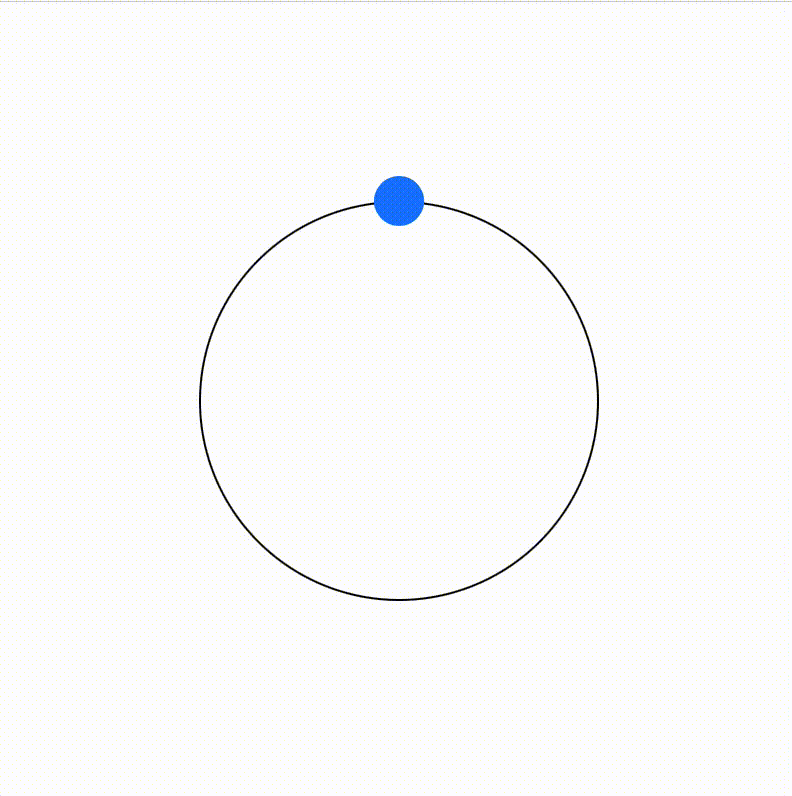Hooray! Much better.

But there is an obvious problem. We are now dealing with a shape — what about any other kind of SwiftUI object? How about adding`Text` that we want to move alongside the circle?

We need a more general solution.

1. # The general solution: AnimatableModifier

This is the real secret of animations in SwiftUI: every animation gets it’s own `AnimatableModifier`. Here is the `AnimatableModifier` for the circle animation:

We see the same `animatableData` as before. But now instead of a `path` method for a shape, we implement the `body` method that returns some modified view. In this case, we are using the angle to update the position of the view.

To apply it, we just use the `.modifier` modifier:

``````.modifier(CircleAnimation(angle: angle, radius: radius))
``````

We can add this modifier to anything —any SwiftUI object. Then when we change the `angle` in the `withAnimation` block, the modifier will control how the changing angle translates into changing position (or whatever other modifiers are being changed).

For example, we can create a `ZStack` involving both the `Circle` and `Text` :

``````ZStack {
Circle()
.frame(width: 50, height: 50)
.foregroundColor(.blue)
``````
``````   Text("Hello!")
.offset(x: 80)
.font(.system(size: 24))
}
``````

Here is the result and complete code: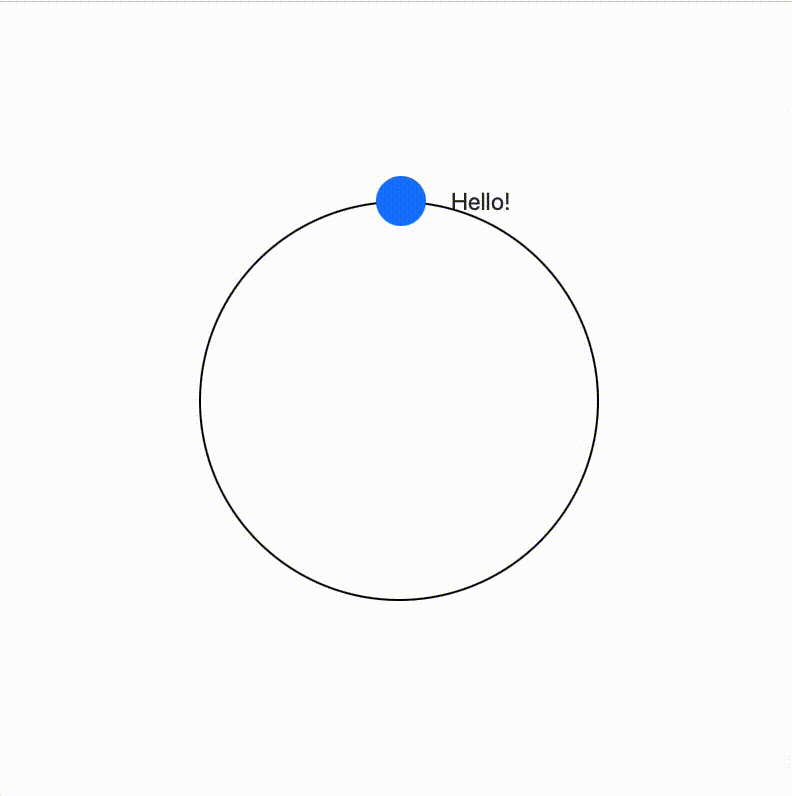# Final thoughts

We worked our way from simple `.animation` modifiers to inheriting from the `AnimatableModifier` protocol. I really prefer this final approach, since it is not only more powerful in letting you control the animations, but also more readable — what data is really being animated is clearly defined in the `animatableData` property. Additionally, it’s reusable — it can be applied to any SwiftUI object to create consistent animations — which to me makes it worth the extra code.